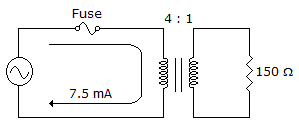# Electronics - Transformers

### Exercise :: Transformers - Filling the Blanks

1.

To ensure a high coefficient of coupling, all fixed transformers are usually wound on a(n) _____________.

 A. EI core B. iron core C. common core D. CI core

Explanation:

No answer description available for this question. Let us discuss.

2.The reflected resistance is ___ as seen by the source in the given circu.

 A. 150B. 300C. 600D. 2.4 kExplanation:

No answer description available for this question. Let us discuss.

3.The secondary current is _____ in the given circuit.

 A. 1.9 mA B. 7.5 mA C. 30 mA D. 60 mA

Explanation:

No answer description available for this question. Let us discuss.

4.

Making the load resistance appear the same as the source's internal resistance is called _____.

 A. maximum power transfer B. reflected resistance C. impedance matching D. reflected load

Explanation:

No answer description available for this question. Let us discuss.

5.

A transformer turns ratio of ________ will be needed to match an output impedance of 5 kto an 8speaker.

 A. 5:1 B. 8:1 C. 25:1 D. 625:1